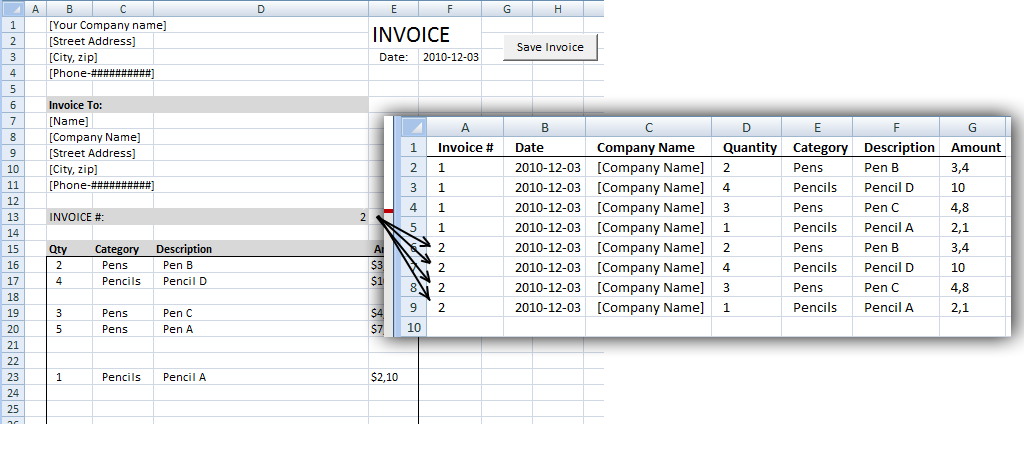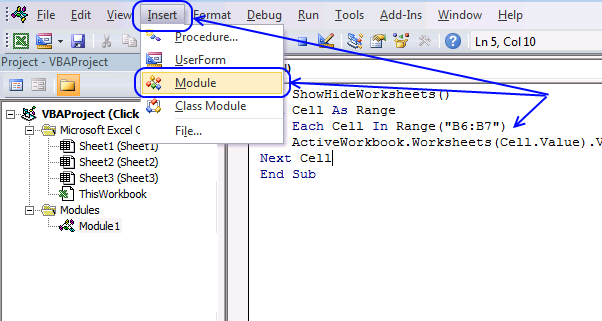Author: Oscar Cronquist Article last updated on January 07, 2019In a previos post:Excel vba: Save invoice data we added/copied data between sheets. This post describes how to overwrite existing values with new values.

If invoice number already exists, a message box asks if you want to overwrite old values with new values.

VBA code:

```Sub Button2_Press with left mouse button on()
Dim rng As Range
Dim i As Long
Dim a As Long
Dim rng_dest As Range
Application.ScreenUpdating = False
'Check if invoice # is found on sheet "Invoice data"
i = 1
Do Until Sheets("Invoice data").Range("A" & i).Value = ""
If Sheets("Invoice data").Range("A" & i).Value = Sheets("Invoice").Range("D13").Value Then
If MsgBox("Overwrite invoice data?", vbYesNo) = vbNo Then
Exit Sub
Else
Exit Do
End If
End If
i = i + 1
Loop
i = 1
Set rng_dest = Sheets("Invoice data").Range("D:G")
'Delete rows if invoice # is found
Do Until Sheets("Invoice data").Range("A" & i).Value = ""
If Sheets("Invoice data").Range("A" & i).Value = Sheets("Invoice").Range("D13").Value Then
Sheets("Invoice data").Range("A" & i).EntireRow.Delete
i = 1
End If
i = i + 1
Loop
' Find first empty row in columns D:G on sheet Invoice data
Do Until WorksheetFunction.CountA(rng_dest.Rows(i)) = 0
i = i + 1
Loop
'Copy range B16:I38 on sheet Invoice
Set rng = Sheets("Invoice").Range("B16:E38")
' Copy rows containing values to sheet Invoice data
For a = 1 To rng.Rows.Count
If WorksheetFunction.CountA(rng.Rows(a)) <> 0 Then
rng_dest.Rows(i).Value = rng.Rows(a).Value
'Copy Invoice number
Sheets("Invoice data").Range("A" & i).Value = Sheets("Invoice").Range("D13").Value
'Copy Date
Sheets("Invoice data").Range("B" & i).Value = Sheets("Invoice").Range("F3").Value
'Copy Company name
Sheets("Invoice data").Range("C" & i).Value = Sheets("Invoice").Range("B8").Value
i = i + 1
End If
Next a
Application.ScreenUpdating = True
End Sub
```

1. Press Alt-F11 to open visual basic editor
2. Press with left mouse button on Module on the Insert menu
3. Copy and paste above vba code
4. Exit visual basic editorThe macro displayed in the picture above is not used in this article.

Note: Save your workbook with the file extension *.xlsm (Macro-enabled workbook) to save the macro to your workbook. If you don't the macro will be gone the next time you open your workbook.

### Get the Excel fileEdit-invoice-data.xls